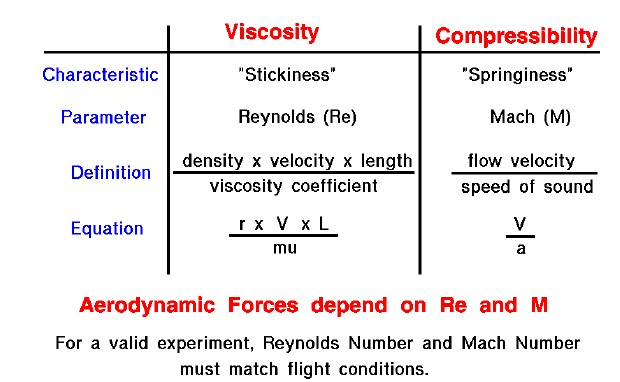# Similarity ParametersThe Wright brothers approached aerodynamics in a thorough, practical, experimental way. From their writings, it is evident that they were very concerned about accurately determining the lift and drag of their aircraft. But they were more practical engineers than theoreticians and many of factors which affect lift and drag are better understood today than they were by the Wright brothers. For a more complete understanding, we present here a page which describes the modern theories of viscosity and compressibility effects on any moving object. The Wright aircraft were certainly subjected to viscous effects, since this causes boundary layer separation and wing stall, which the brothers experienced in flight, and has a large effect on overall aircraft drag. Fortunately, the Wright aircraft flew at very low velocities and were not subject to compressibility effects.

## Aerodynamic Forces

As an object moves through the air, the air molecules near the object are disturbed and move around the object. Aerodynamic forces are generated between the gas and the object. The magnitude of these forces depend on the shape of the object, the speed of the object, the mass of the air going by the object and on two other important properties of the air; the viscosity, or stickiness, of the air and the compressibility, or springiness, of the air. To properly model these effects, aerodynamicists use similarity parameters, which are ratios of these effects to other forces present in the problem. If two experiments have the same values for the similarity parameters, then the relative importance of the forces are being correctly modeled. Representative values for the properties of air are given on another page, but the actual value of the parameter depends on the state of the gas and on the altitude.

## Boundary Layer

Aerodynamic forces depend in a complex way on the viscosity of the air. As an object moves through the air, the air molecules stick to the surface. This creates a layer of air near the surface (called a boundary layer) which, in effect, changes the shape of the object. The flow turning reacts to the boundary layer just as it would to the physical surface of the object. To make things more confusing, the boundary layer may lift off or “separate” from the body and create an effective shape much different from the physical shape. And to make it even more confusing, the flow conditions in and near the boundary layer are often unsteady (changing in time). The boundary layer is very important in determining the drag of an object. To determine and predict these conditions, aerodynamicists rely on wind tunnel testing and very sophisticated computer analysis.

## Reynolds Number

The important similarity parameter for viscosity is the Reynolds number. The Reynolds number expresses the ratio of inertial (resistant to change or motion) forces to viscous (heavy and gluey) forces and is given by the equation Re = velocity x density x characteristic length/viscosity coefficient. If the Reynolds number of the experiment and flight are close, then we properly model the effects of the viscous forces relative to the inertial forces. If they are very different, we do not correctly model the physics of the real problem and will predict an incorrect lift.

Aerodynamic forces also depend in a complex way on the compressibility of the air. As an object moves through the air, the air molecules move around the object. If the object passes at a low speed (typically less than 200 mph) the density of the fluid will remain constant. But for high speeds, some of the energy of the object goes into compressing the fluid and changing the density, which will alter the amount of resulting force on the object. This effect becomes more important as speed increases. Near and beyond the speed of sound (about 330 m/s or 700 mph), shock waves are produced that affect both the lift and drag of an object. Again, aerodynamicists rely on wind tunnel testing and sophisticated computer analysis to predict these conditions.

## Mach Number

The important similarity parameter for compressibility is the Mach number, the ratio of the velocity to the speed of sound. So it is completely incorrect to measure a lift coefficient at some low speed (say 200 mph) and apply that lift coefficient at twice the speed of sound (approximately 1400 mph, Mach = 2.0). The compressibility of the air will alter the important physics between these two cases.

The effects of compressibility and viscosity on lift are contained in the lift coefficient and the effects on drag are contained in the drag coefficient.

Provide feedback